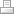# Lecture Commutative Algebra

Modules over commutative rings occur almost everywhere in Mathematics. The first common examples are groups seen as modules over the ring of integers. Modules behave more richer than vector spaces, for example a module over a ring does not need to have a basis anymore.  To understand ring extensions, Dedekind rings and group rings it needs the understanding of modules.
As an application of ring theory we introduce a connection to algebraic geometry: We will finish the course in introducing Gröbner basis and the Buchberger algorithm for finding such basis.

Course TaskTo learn the basic notions and theorems for algebraic objects over commutative rings. The course is about

* modules (flat, free, projective, injective)

* tensor products of modules,

* localization

* integral ring extensions

* completions and Hensel’s Lemma

* röbner basis

The final mark will be computed in the following way: 50% homework and 50% final exam

Literature: 1) David Eisenbud, Commutative Algebra with a view towards Algebraic Geometry

2) Atiyah M.F. and Macdonald I.G. “Introduction to Commutative Algebra"

Problems to present to the tutors:

Week (20.9--): Problem 2 (Sheet 1)

Week (27.9--): Problem 2.4

Week (11.10.-) Problem 4.2 (or easier 4.3)

Week (18.10-) Problem 5.1

Week (25.10-) Problem 5.4

Week (1.11-) Talk about flatness, Problem 7.4

Week (8.11-) Problem 8.2

Week (22.11-) Problem 10.2

Week (29.11.-) Problem 11.1

Week (6.12.-) Problem 12.2

Week (20.12.-) Problem 13.4

Exercise sheets: Sheet 1 Sheet 2 Sheet 3 Sheet 4 Sheet 5 Sheet 6 Sheet 7

Sheet 8 (8.2 has been changed) Sheet 9 Sheet 10 Sheet 11 Sheet 12 Sheet 13

Lecture notes can be found here.

Prof. Daniel Skodllerack

Shanghaitech University

Institute for Mathematical Sciences,  Room S413

Office hours:

Mondays  19:00-20:00 and Sundays 19:00-20:00Print | Sitemap